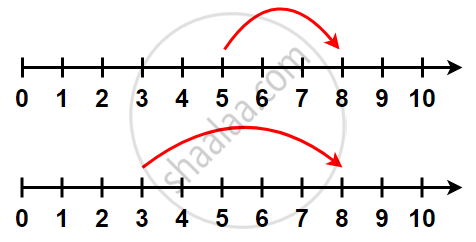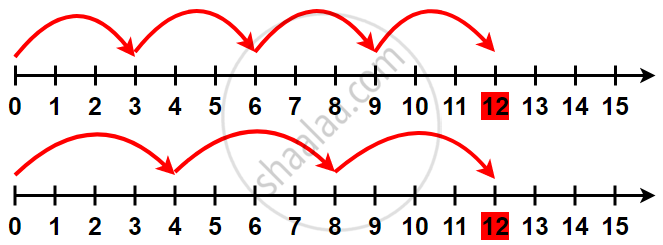Tamil Nadu Board of Secondary EducationSSLC (English Medium) Class 6th

# Properties of Whole Numbers - Commutativity Property of Whole Number

## Notes

### Commutative Property of Whole Numbers:In both cases, we reach 8. 5 + 3 is the same as 3 + 5.
You can add two whole numbers in any order.
We say that addition is commutative for whole numbers. This property is known as the Commutativity of addition.

## 2. Commutativity of multiplication:You will observe that 3 × 4 = 4 × 3.
You can multiply two whole numbers in any order.
We say multiplication is commutative for whole numbers.

## 3. Commutativity of Subtraction:

a - b ≠ b - a
a = 3, b = 5
a - b = b - a
3 - 5 = 5 - 3
- 2 ≠ 2

Thus, Subtraction is not commutative for whole numbers.

## 4. Commutativity of Division:

a ÷ b ≠ b ÷ a
a = 9 and b = 3

9/3 = 3/9

3 ≠ 0.333.

Thus, Division is not commutative for whole numbers.

If you would like to contribute notes or other learning material, please submit them using the button below.

### Shaalaa.com

Commutative Property of Whole Numbers [00:08:59]
S
0%